Open in App
Not now

# Less.js Options Strict Units

• Last Updated : 31 Jan, 2023

Less stands for Leaner Style Sheets is a CSS preprocessor and is a backward-compatible language extension for CSS. Less provides many features such as variables, in the development, it is common to see the same value repeated again and again so in this case variables help to reuse the code and provide more such options to make it easy to handle the code. It is a JavaScript tool that converts Fewer styles to CSS styles. In this article, we will discuss Strict Unit options:

• strictUnits: false: Basically by default strictUnits is set to false so, in that case, Less first performs a mathematical operation and then decides the respective unit, or in other words Fewer attempts to guess the output unit after the mathematical operation.
• strictUnits: true: But when the strictUnits is set to on/true, it will give an error because, for example, if we multiply length by length, it gives the area, but CSS does not specify the area. So Less assume that the user meant for one of the value to be a value and not a unit of length.

We will explore all the above approaches through examples. Before coming to the examples first we have to install the LESS, to install the Less follow the commands below:

Step 1: Create a Folder named ‘Less’:

`mkdir Less Strict Units`

Step 2: Move to the created folder and initialize npm init:

`npm init`

Step 3: Install Less Globally using ‘-g’:

`npm install less -g`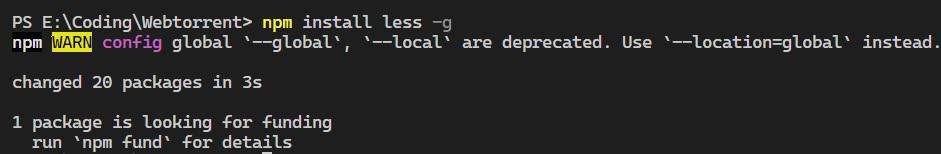Step 4: Create a file named main.less and index.html files so that we can show the output. In this file, we will code it.

Project Structure: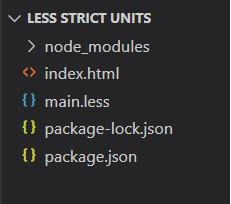Example 1: In this example, Less attempts to guess the output unit after the calculation is done when the strictUnits is set to false. By default, the strictUnits is set to strictUnits: off or strictUnits:false.

index.html

## HTML

 ` ` `<``html` `lang``=``"en"``> ` ` `  `<``head``> ` `    ``<``title``>Less Strict Mode off ` `    ``<``link` `rel``=``"stylesheet"` `href``=``"main.css"``> ` ` ` ` `  `<``body``> ` `    ``<``div` `class``=``"box"``>Box ` ` ` ` `  ``

main.less

## Javascript

 `.box{ ` `    ``height: 20px*20px; ` `    ``width: 20px*20px; ` `    ``border: 2px solid red; ` `}`

To get the output using the strictUnits: ‘false’ follow the command below. Here main.less is the current file that is already present and main.css is the file that is created after compiling main.less.

`lessc --strict-units=false main.less main.css`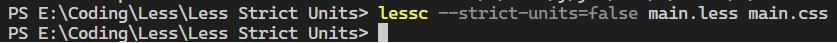After that, a new file is get created which is main.css, and in this case, things are not clearly right a length is multiplied by length which gives an area but here the CSS does not support areas so it just performs math operations and then gives the unit and outputs 400px as length and width because here the strictUnits is set to strictUnits: ‘false’.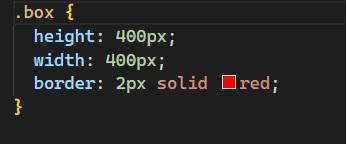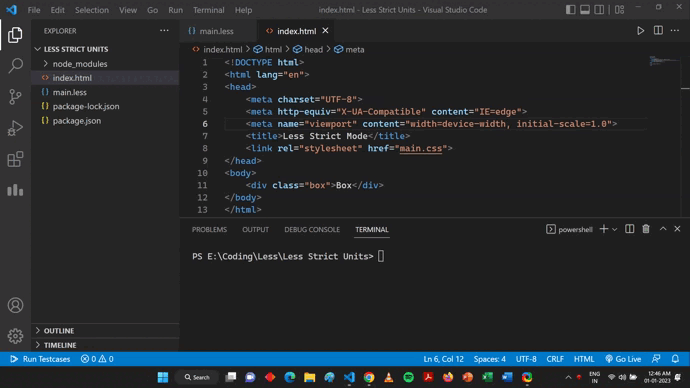Example 2: In this example now we turn on the strict units option and with the strict option ‘on’ we assume that there is a bug in the calculation and throw an error.

index.html

## HTML

 ` ` `<``html` `lang``=``"en"``> ` `<``head``> ` `    ``<``title``>Less Strict Mode On ` `    ``<``link` `rel``=``"stylesheet"` `href``=``"main.css"``> ` ` ` `<``body``> ` `    ``<``div` `class``=``"box"``>Box ` ` ` ` `

main.less

## Javascript

 `.box{ ` `    ``height: 20px*20px; ` `    ``width: 20px*20px; ` `    ``border: 2px solid red; ` `} `

Output: To get the output using the strictUnits: ‘true’ follow the command below:

Here main.less is the current file that is already present and main.css is the file that is created after compiling main.less.

`lessc --strict-units=true main.less main.css`

In this case, a new file will not get created which is main.css because the file is getting compiled with the strictUnits mode on, and we assume that there is a bug in the calculation and throw an error.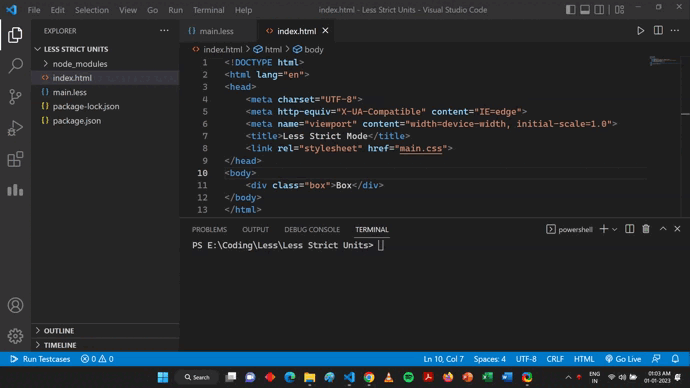My Personal Notes arrow_drop_up
Related Articles